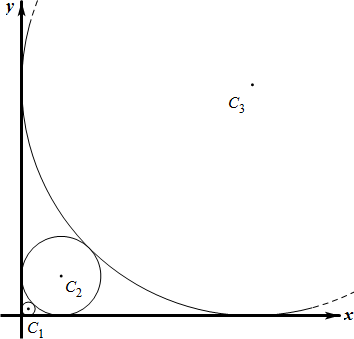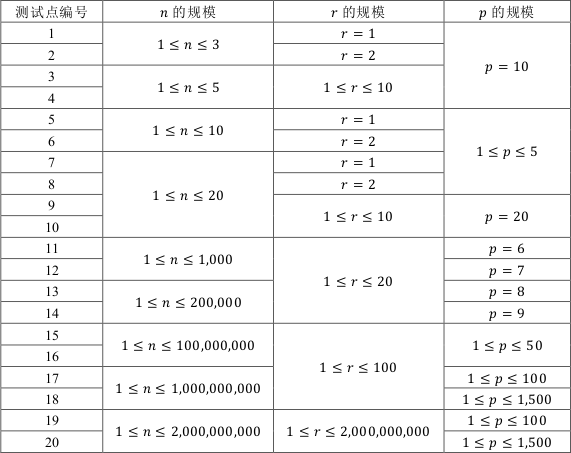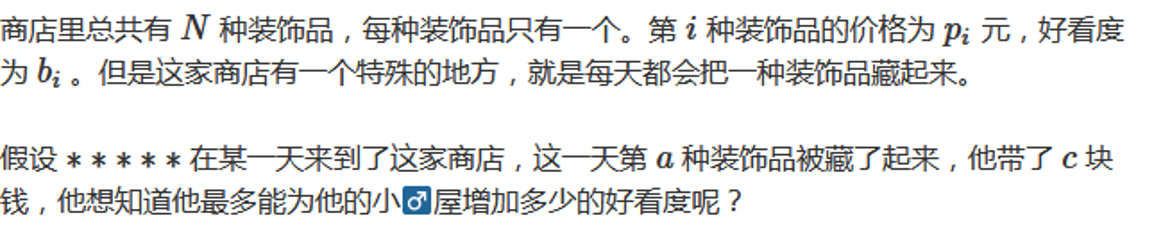# YZOJ P3754 Gab数列

• ### 题目描述

1，$$\forall 1\le i\le m$$ 满足 $$b_i\in\mathbf{N^*}$$ 且 $$1\le b_i\le n$$

2，$$\sum_\limits{i=1}^{m}b_i\le k$$

…

# YZOJ P2417 [FJWC2016][CF79-D]翻转硬币

• ### 题目描述

$$n$$ 枚硬币正面朝上摆成一排，给定 $$a_1, a_2, \cdots, a_m$$，每次操作可以任意选择一个 $$a_i$$，并翻转连续 $$a_i$$ 个硬币

Source: CF79-D

# YZOJ P2697 画圆

• ### 题目描述

$$Alkri$$ 想在平面直角坐标系的第一象限中依次画 $$n$$ 个与两坐标轴均相切的圆，其中，第 $$1$$ 个圆的半径为 $$r$$，之后的每个圆都比上一个圆大，且与上一个圆相切，也就是说，对所有整数 $$2 \leq i \leq n$$，第 $$i$$ 个圆的半径大于第 $$i-1$$ 个圆的半径且与第 $$i-1$$个圆相切。• ### 数据规模与约定…

# YZOJ P3314 计算器

• ### 题目描述

< 表达式 > ：  < 运算数 1>< 运算符 1>< 运算数 2>< 运算符 2> $$\cdots$$ < 运算符 $$k-1$$>< 运算数 $$k$$>（ $$k$$ 为正整数）

• ### 数据规模与约定

$$1 \leq \left| s \right| \leq 10^4$$

…

# YZOJ P2319 宇宙图书馆

• ### 输入格式

Add t x：添加一套共 $$x$$ 本书（$$x$$ 为正整数），这一套书的出版年份均为 $$t$$（单位：宇宙年）；

Remove t：删除所有出版年份小于 $$t$$ （单位：宇宙年）的书，并输出被删除的书的数量；

Count a b：统计出版年份（单位：宇宙年）在 $$a, b$$ 之间（含 $$a, b$$，保证 $$a \leq b$$ ）的书的数量，并输出；

List x：将所有书按出版年份从大到小（也就是从新到旧）顺序列出，但由于输出可能较多，这里只要求输出列表中给定的第 $$x$$ 本书的出版年份（如果不存在，输出 No）；

Quit：退出系统，结束程序。

# YZOJ P3669 [USACO2008 Mar]土地购买

• ### 题目描述

FJ 希望买下所有的土地，但是他发现分组来买这些土地可以节省经费。他需要你帮助他求出最小的经费。

…

# YZOJ P3129 [校内训练20170627]跳格子

• ### 题目描述

1， $$p_1=s ，p_n=t$$；

2， 对任意 $$2 \leq i \leq n-1$$，或者 $$(p_i-1<p_i) \land (p_i+1<p_i)$$ ，或者 $$(p_i-1>p_i) \land (p_i+1>p_i)$$ 。

• ### 数据规模与约定…

# [CF868-F] Yet Another Minimization Problem

（其他样例见原题

• ### 数据范围

$$Source$$: CF868-F

# [2017-2018 Petrozavodsk WC] J. Subsequence Sum Queries

• ### 题目描述…